1800-1023-196

+91-120-4616500

# Relation of Osmotic Pressure with Different Colligative Properties

## Relation of Osmotic Pressure with Different Colligative Properties

Osmotic pressure is related to relative lowering of vapour pressure, elevation of boiling point and depression of freezing point according to the following relations,

(a)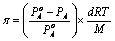(b)(c)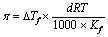In the above relations, p = Osmotic pressure; d = Density of solution at temperature T; R = Universal gas constant;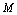= Mol. Mass of solute;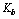= Molal elevation constant of solvent;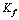= Molal depression constant of solvent

(v) Isotonic, Hypertonic and Hypotonic solutions

(a) Isotonic or iso-osmotic solutions:Two solutions of different substances having same osmotic pressure at same temperature are known as isotonic solutions.

For isotonic solutions,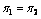Primary Condition …..(i)Also,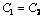or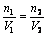or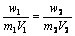…..(ii)

Eq. (ii) holds good only for those solutes which neither possess the tendency to get associate nor dissociate in solution, e.g.,

Urea and glucose are isotonic then,andUrea and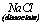are isotonic then,but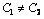Urea and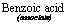are isotonic then,but(b) Hypertonic and hypotonic solution:The solution which has more osmotic pressure than the other solution is called as hypertonic solution and the solution which has lesser osmotic pressure than the other is called as hypotonic solution.

The flow of solvent is always from lower osmotic pressure to higher osmotic pressure i.e. from hypotonic to hypertonic solution.

## NEET & AIIMS Exam Sample Papers

 AIIMS SAMPLE PAPERS View More NEET SAMPLE PAPERS View More